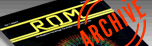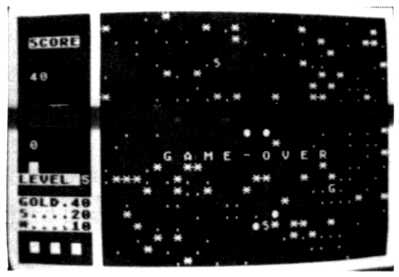`ROM MAGAZINE ISSUE 2 — OCTOBER/NOVEMBER 1983 / PAGE 17`

 Star Bomb by Toung Tran     The year is 2122 and you as the last star pilot must destroy each of the stars by using your plasma cannon mounted on the front of your space craft. While you're trying to destroy the stars one to five Alien space ships (Depending on level) try to crash into you, thus destroying one of your three lives. Unfortunately, you are unable to destroy these space ships because they have a special cloaking device which makes it impossible to hit them. The only thing that can be done is to avoid them. There are also different types of ore and minerals that can be picked up for valuable points. They are D-Diamonds, P-Plutonium, G-Gold, and S-Silver. These will appear randomly on the screen as the game progresses.     The game uses the Atari's character set to draw the space ships. These ships are poked onto the screen and then moved around through poking new positions. The ship moves with the joystick and fires by pushing the red button. The game takes about a minute to intialize as it randomly plots the different stars. Remember don't touch the stars.10 DIM P\$(3),A(5),X(5),Y(5),F(5),ROBOT(5):COLOR 2 20 SCREEN=PEEK(88)+256*PEEK(89) 30 GOTO 9000 39 REM --- CONTROL-SHIPS--- 40 S=STICK(0) 42 IF S=15 THEN RETURN 45 SOUND 0,l50,10,10:POKE P,A(0):SOUND 0,0,0,0 50 IF S=13 THEN M=l:SHIP=87:F=40:G0SUB 300 60 IF S=14 THEN M=-l:SHIP=88:F=-40 :GOSUB 300 70 IF S=7 THEN M=1:SHIP=65:F=1:GOSUB 300 80 IF S=11 THEN M=-1:SHIP=68:F=- 1:GOSUB 300 85 P=SCREEN+X+40*Y:A(0)=PEEK(P) 87 IF A(0)=84 THEN MAN=MAN-2:J=P:GOSUB 5000:RETURN 88 IF A(0)<>0 AND A(0)<>14 THEN GOSUB 800 90 POKE P,SHIP:RETURN 99 REM ----INITIALIZE--- 100 GOSUB 200 110 H=SCREEN+A+40*B 120 IF PEEK(H)=10 THEN FOR W=50 TO 100 130 IF PEEK(H)=14 THEN SOUND 0,20,10,10:POKE H,10:SOUND 0,0,0,0 140 IF INT(RND(0)*4)=l AND PEEK(H)=0 THEN SOUND 0,100,10,10:POKE H,14:SOUND 0,0,0,0 150 RETURN 200 A=9+INT(RND(0)*30) 210 B=1+INT(RND(0)*22):RETURN 249 REM SET UP SHIPS 250 V=INT(RND(0)*20):ON V GOTO 270,280,280,290,290,290,291,291,291,291 260 F(T)=10:RETURN 270 F(T)=36:RETURN 280 F(T)=48:RETURN 290 F(T)=39:RETURN 291 F(T)=51 RETURN 299 REM CHECK SHIP 300 IF PFEK(P+F)=128 THEN MAN=MAN-2:J=P:GDSUB 5000:RETURN 310 IF PEEK(P+F)=10 THEN MAN=MAN-2:POKE P+F,0:J=P:GOSUE 5000;RETURN 320 IF S=13 OR S=14 THEN Y=Y+M:RETURN 330 IF S=11 OR S=7 THEN X=X+M:RETURN 350 ? "↓(5x)":POKE 85,15:? "G A M E - 0 V E R" 355 IF STRIG(0)<>0 THEN 355 360 ? "↑↑↑ DO YOU WANT TO PLAY AGAIN (Y/N)";:INPUT P\$ 365 IF SCORE>HI THEN HI=SCORE 370 IF P\$="Y" OR P\$="YES" THEN 30 380 IF P\$="N" OR P\$="NO" THEN END 390 GOTO 360 399 REM MOVE ALIEN SHIPS 400 T=T2+INT(RND(0)*(10-T1)):IF T>5 THEN RETURN 403 POKE ROBOT(T),F(T) 405 IF Y(T)>Y THEN Y(T)=Y(T)-1 410 IF Y(T)X THEN X(T)=X(T)-l 430 IF X(T)=39 OR Y(T)<=0 OR (T)>=23 THEN 442 440 ROBOT (T)=SCREEN+X(T)+40*Y*(T):F(T)=PEEK(ROBOT(T)) 442 POKE ROBOT(T),84 445 IF F(T)=65 OR F(T)=68 OR F(T)=87 OR F(T)=88 THEN MAN=MAN-2:J=P:F(T)=0:GOSUB 5000:RETURN 448 IF F(T)<>0 AND F(T)<>10 THEN GOSUB 8000 460 RETURN 469 REM KEEP SCORE 470 SETCOLOR 2,16,1:FOR W=1 to 23:POKE 85,0:?;"(16 inverse spacea)":NEXT W:? "↑(15 times)" 500 ? "↑(8 times)":? "SCORE↓↓"? "↓↓↓→→ HI↓←(4 times)SCORE"? :? HI 501 ? :POKE 85,1:? ;"LEVEL ";LEVEL 502 ? :POKE 85,1:? ;"D...100":POKE 85,1:? ;:P....50":POKE 85,1:? ;"G....40" 503 POKE 85,1:? ;"S....20" 504 POKE 85,1:? ;"*....10":? "":?"    ":?"  ↑" 505 FOR W=1 TO 5 STEP 2:POKE SCREEN+(1+W)+40*21,216:NEXT W 509 SETCOLOR 2,16,1:FOR W=0 TO 39:POKE SCREEN+W,128:NEXT W 510 FOR W=0 TO 24;POKE SCBEEN+40*W,128:POKE SCREEN+8+40*W,128:POKE SCREEN+40*W+39,128:NEXT W 520 FOR W=0 TO 39:POKE SCREEN+W+40*23,128:NEXT W 600 FOR Y=1 TO 22:W1=9:W2=38:W3=1:IF INT(Y/2)=Y/2 THEN W1=38:W2=9:W3=-1 605 FOR X=W1 TO W2 STEP W3:PI=0:IF INT(RND(0)*(60-LEVEL*10))=2 THEN PI=10 610 IF INT(RND(0)*(30-LEVEL*5))=3 THEN PI=14 620 POKE SCREEN+X+40*Y,PI:NEXT X:NEXT Y 690 GOSUB 950:GOTO 9050 800 SOUND 0,48,l0,10:IF A(0)=36 THEN SCORE=SCORE+100 810 IF A(0)=48 THEN SCORE=SCORE+50 820 IF A(0)=39 THEN SCORE=SCORE+40 830 IF A(0)=51 THEN SCOBE=SCORE+20 840 ?" ";SCORE:A(0)=0:RETURN 950 POKE SCREEN+(1+MAN)+40*21,128:GOSUB 200:X=A:Y=B 960 SHIP=88:P=SCREEN+X+40*Y:FOR W=100 TO 50 STEP -1:SOUND 0,W,10,10:POKE P,216:POKE P,88:NEXT W:SOUND 0,0,0,0 970 IF STICK(0)=15 THEN 970 980 POKE P,0:RETURN 3000 J=H:VER=40:HOR=1:O=0 3005 GOSUB 6000 3010 IF O=1 THEN RETURN 3020 IF O=2 THEN J=P2:MAN=MAN-2:GOSUB 5000:A(0)=0:RETURN 3080 GOTO 3005 5000 FOR W=40 TO 70:SOUND 0,W,12,12:NEXT W:SOUND 0,0,0,0:COUNT=0:VER=40:HOR=1:O=0:A(0)=0 5005 GOSUB 6000 5010 IF O=1 THEN 5040 5020 COUNT=COUNT+1:IF COUNT=3 THEN 5040 5030 GOTO 5005 5040 IF U=1 THEN RETURN 5050 IF MAN<1 THEN 350 5060 FOR Q=1 TO 200:NEXT Q 5070 GOSUB 950:RETURN 6000 POKE J,0 6005 A(1)=PEEK(J+VER):A(2)=PEEK(J-VER):A(3)=PEEK(J+HOR):A(4)=PEEK(J-HOR) 6020 POKE J+VER,76:POKE J-VER,76 6030 POKE J+HOR,76:POKE J-HOR,76 6040 IF A(1)=128 OR A(2)=128 OR A(3)=128 OR A(4)=128 THEN O=1 6041 IF A(1)=65 OR A(1)=68 OR A(1)=87 OR A(1)=88 THEN O=2:P2=J+VER 6042 IF A(2)=65 OR A(2)=68 OR A(2)=87 OR A(2)=88 THEN O=2:P2=J-VER 6043 IF A(3)=65 OR A(3)=68 OR A(3)=87 OR A(3)=88 THEN O=2:P2=J+HOR 6044 IF A(4)=65 OR A(4)=68 OR A(4)=87 OR A(4)=88 THEN O=2,P2=J-HOR 6050 POKE J+VER,A(1):POKE J-VER,A(2) 6060 POKE J+HOR,A(3):POKE J-HOR,A(4} 6070 VER=VER+40:HOR=HOR+1:RETURN 7000 IF PEEK(P)=87 THEN N=40:D=93 7010 IF PEEK(P)=88 THEN N=-40:D=92 7020 IF PEEK(P)=65 THEN N=1:D=95 7030 IF PEEK(P)=68 THEN N=-1:D=94 7035 C=1:P1=P 7040 A(5)=PEEK(P1+N*C):IF A(5)=128 OR A(5)=84 THEN RETURN 7045 IF A(5)=10 THEN SCORE=SCORE+10:J=P1+N*C:U=1:GOSUB 5000:U=0? "↑";SCORE:RETURN 7060 POKE P1+N*C,D 7066 If C>2 THEN GOSUB 40:GOSUB 400 7070 POKE P1+N*C,A(5) 7080 C=C+1:GOTO 7040 8000 REM EXAMINE ALIEN 8010 IF F(T)=84 OR F(T)=92 OR F(T)=93 OR F(T)=94 OR F(T)=95 OR F(T)=51 OR F(7)=39 OR F(T)=36 OR F(T)=48 THEN F(T)=0 8020 IF F(T)=14 THEN GOSUB 250 8030 RETURN 9000 REM --- MAIN-PROGRAM--- 9010 GRAPHICS 18:MAN=5:A(0)=0:SCORE=0:LEVEL=1 9012 POSITION 4,1:? #6;"*star bomb*":POSITION 4,1:? #6;"by toung tran" 9013 POSITION 6,4:? #6;"LEVEL":POSITION 1,8:?#6;"PUSH START TO BEGIN" 9014 POSITION 2,6:? #6;"SELECT FOR LEVEL" 9015 POSITION 11,4:? #6;LEVEL 9016 L=PEEK(53279):IF L=7 THEN 9016 9017 IF L=5 THEN LEVEL=LEVEL+1;IF LEVEL>5 THEN LEVEL=1 9018 IF L=6 THEN 9020 9019 GOTO 9015 9020 Tl=10-LEVEL:T2=6-LEVEL:GRAPHICS 0:SETCOLOR 2,16,1 9030 IF LEVEL<=2 THEN T1=7-LEVEL 9040 FOR E=l TO 5:GOSUB 200:X(E)=A:Y(E)=B:ROBOT(E)=SCREEN+X(E)+40*Y(E):F(E)=0:NEXT E:GOTO 470 9050 GOSUB 40 9070 IF STRIG(0)=0 THEN FOR W=10 TO 20:SOUND 0,W,10,10:NEXT W:SOUND 0,0,0,0.GOSUB 7000 9080 GOSUB 400:GOSUB 100 9100 GOSUB 400 9200 GOTO 9050